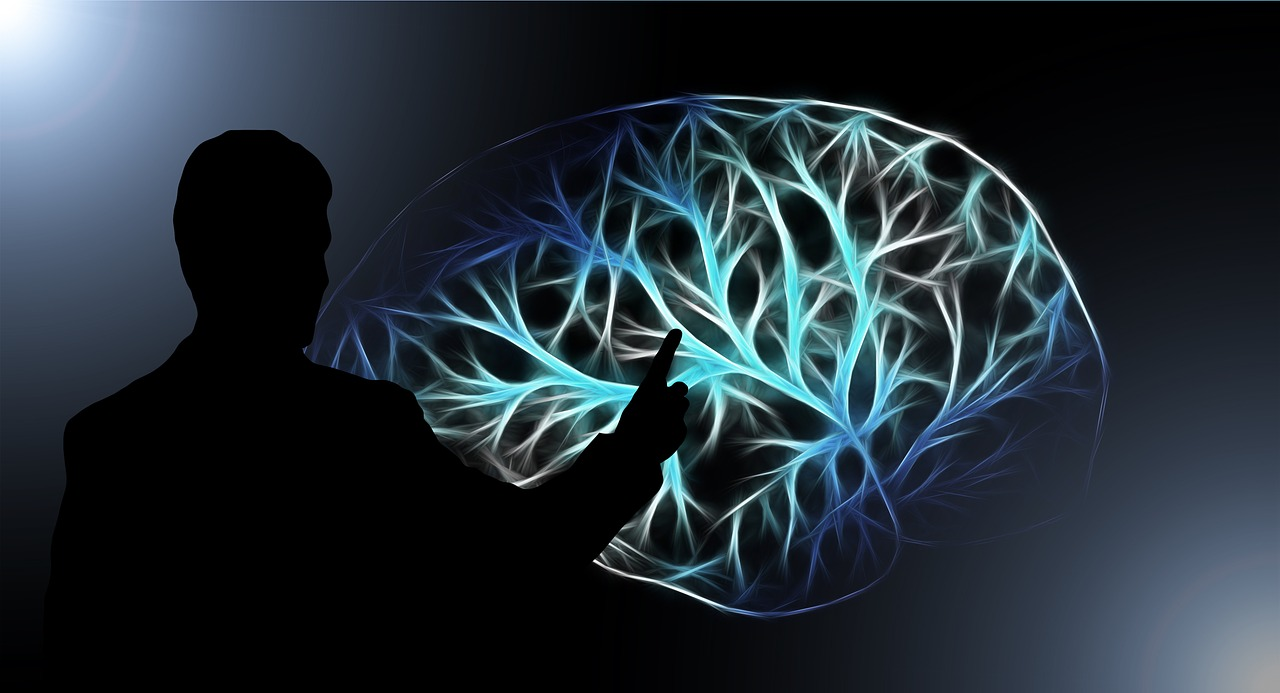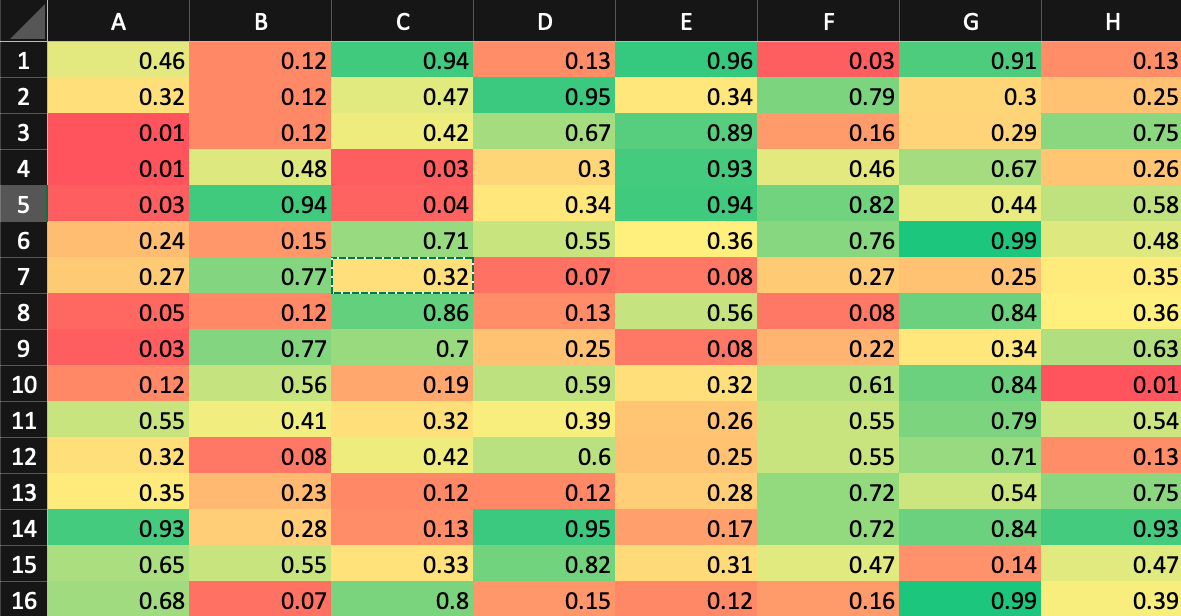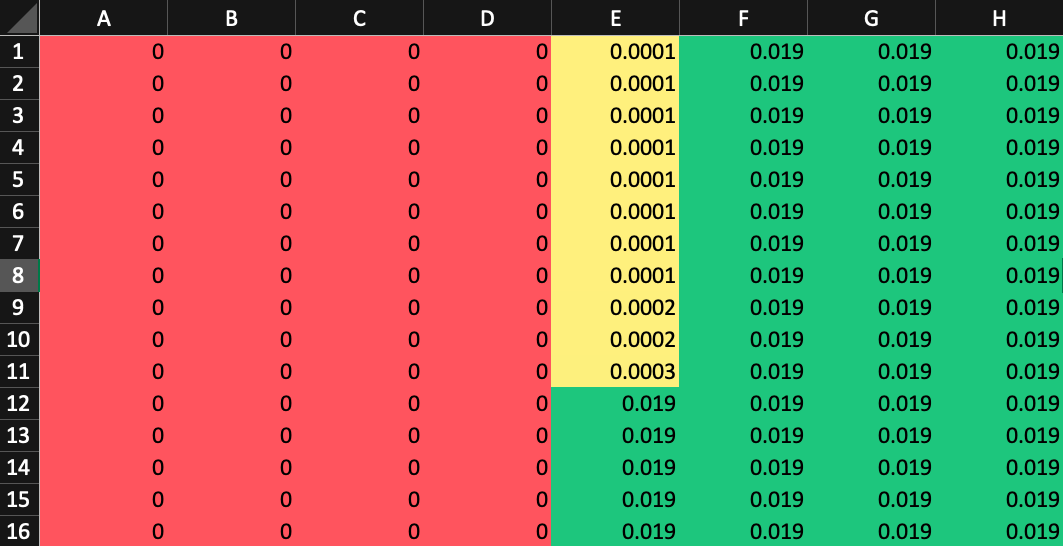Eshan Chatty — Published On September 30, 2020 and Last Modified On October 5th, 2020

This article was published as a part of the Data Science Blogathon.

## Introduction

Yesterday, my brother broke an antique at home. I began to search for FeviQuick (a classic glue) to put it back together. Given that it’s one of the most misplaced items, I began to search for it in every possible drawer and every untouched corner of the house I hadn’t been to in the past 3 months. I gave up the search after an hour – the FeviQuick was nowhere to be found. I narrowed down the places to look and began to search again, only to find it pressed under three books!

But what does this story have to do with machine learning models? Let me explain how I thought through this versus how a machine learning model will think it through.## The Human Mind vs. The Machine – Problem Solving

I would make a list of all the possible places it could be (data). My brain would automatically prioritize which places to search thoroughly. This could be considered as assigning a probability to each of the places (prior). Without yielding any results after my first search, I started thinking about all the probable places. My mother thought she had last seen it in my bedroom (new data). I now thought about the likelihood of where the object could be found, given it was in my bedroom (Mind).

In this whole thinking process, my mind is assigning new probabilities to each of these places (posteriori). Basically, my value of probability is not constrained to one value, but a range of values. This is exactly how Bayesian probabilities are ranged – not a single value, but a distribution of values.

Now for the machine. We input the data containing all the places it was to possibly find it. Each place of finding the object would be equally likely for the model, as the machine knows no such thing called bias, nor does it have any prior training data to refer to.

Now that the machine is unable to get any result, hence it assigns zero value to each of the probabilities present. Even if we taught the machine to only consider a few places, it would give each place an equally likely probability that would not help our case. Here, we can see that the values of the probability are confined to a single value. The machine assigns the value zero if the object isn’t found, as it gives no room to the probability that it could’ve made an error (all machines are prone to error ).

## Machine Learning in the Real World

Consider this being applied to a real-life problem, where there has been a kidnapping and we try to narrow down the possibilities where the kidnapper could’ve taken the person.This is the Prior knowledge we may have, and hence depicting the likelihood of finding the kidnapper given he is in that square.

As humans, we know that no place can be ruled out because first of all, the kidnapper need not be stationary and there is always a possibility that he could be hidden in a place we have no whereabouts of. Hence, we make a probability of where he could be, based on the type of buildings present at each block. A higher probability has been given to those areas which are shady and remotely located. This is called the likelihood.

Now, let’s say we get a tip from a person that he surely saw the person go straight from E13 block ahead? Then our probabilities would change as follows:

Here, we can see that there has been a shift in the alignment of probabilities. The cells towards the right of block E12 have gotten a rise in the green shade. This is also sometimes referred to as the posterior probability. The likelihood has been multiplied with a value E such that the posterior value of the probability has been updated in those regions. This value E is called the evidence.

Despite the information, it has not assigned zero probability to the blocks before E12, because it does take uncertainty into account.

This hierarchy can be applied as a chain of events, with the evidence being updated as soon as new information is fed to it. The posterior for the previous situation will become the likelihood for the updated situation. Let us give a formula to what we’ve been visualizing:

For a machine learning model, such as logistic regression, or even for a multi-layer perceptron, it would be impossible to train these models without considering the Bayesian analogy. For a machine learning model, it would assign values that would look something like this:

Now, let us see how a machine learning model would react to new information being added as before, i.e the kidnapper had been sighted going towards the right of E12:Here the model has very less representation and shows too much bias and dependency on the data

Can you see how biased the model has become? It has straightaway assigned a value of zero to places before E12. This seems rather inconvenient as it does not take into account the factors that the information or data provided might be wrong, hence the model might already be looking in the wrong direction and wasting time.

This is because the models follow the frequentist approach, where, if the item is not found, its probability will be zero regardless of any other conditions specified (the kidnapper may have traveled to the left of block E12 or the informant may be wrong).

## End Notes

So, the Bayesian approach is one that has a personal perspective. This application can prove to be very important in the real-world and the personal assumptions we make are all deemed right if they’re done in a proper mathematical environment.

The frequentist approach, no matter how statistically strong it might be, just cannot take on real-life applications because it has no space for uncertainty as well as the unpredictability of the probability of an outcome.

Since neural networks and machine learning models do not incorporate Bayesian probability, A/B tests and real-world situations often make use of Monte Carlo simulation and Markov models.